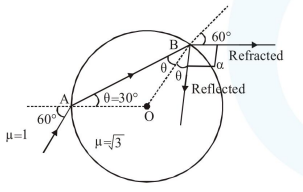# A light ray enters a solid glass sphere of refractive index

Question:

A light ray enters a solid glass sphere of refractive index $\mu=\sqrt{3}$ at an angle of incidence $60^{\circ}$. The ray is both reflected and refracted at the farther surface of the sphere. The angle (in degrees) between the reflected and refracted rays at this surface is

Solution:By Snell's law at $\mathrm{A}$ :

$1 \times \sin 60^{\circ}=\sqrt{3} \times \sin \theta$

$\frac{\sqrt{3}}{2}=\sqrt{3} \sin \theta$

$\sin \theta=\frac{1}{2} \Rightarrow \theta=30^{\circ}$

So at B :

$\theta+60^{\circ}+\alpha=180^{\circ}$

$30^{\circ}+60^{\circ}+\alpha=180^{\circ}$

$\alpha=90^{\circ}$